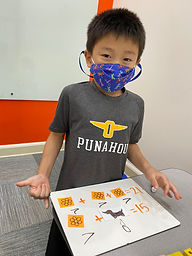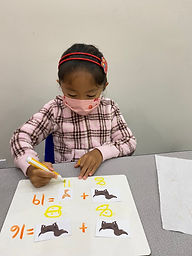Ms. Brianne

Target 1​

Lesson Type:

Continuation

Algebra

:

Variable

Solve addition and subtraction problems where a symbol is used to represent an unknown quantity.

1:

Informal introduction to variables.

2:

Explain that symbols can be anything: a question mark, a blank space, a color, a shape. The symbol is just an indication of what needs to be solved.

3:

Look closely at the problem to determine what needs to be solved, it is not always after the =.

2nd

Vocabulary:

Activities:

• Students were given addition or subtraction equations with two picture variables.
• Students used the numbers in the equations to figure out the value of one variable. Then they used that value to help them solve for the other variable.Home Exploration

Guiding Questions:Absent Students:

Target 2

:

3rd

Vocabulary:

Volume

Activities:

Students filled 3D shapes with blocks and counted them to find the figure's volume.

• Students used blocks to copy figures and counted the number of blocks to find the volume.
• Students created different arrangements of blocks with the same number of blocks to help them understand that figures can look different, but have the same volume.Home Exploration

Guiding Questions:Target 3

:

Vocabulary:

Activities:Home Exploration